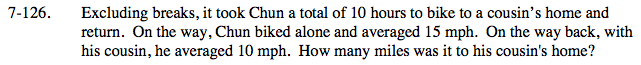### Home > PC > Chapter 7 > Lesson 7.3.3 > Problem7-126

7-126.(rate)(time) = distance

Since the distance to the house is the same as the distance from the house:

$\left(15 \frac{\text{miles}}{\text{hour}}\right)(t\text{ hours})=\left ( 10 \frac{\text{miles}}{\text{hour}}\right )(10 \text{ hours }-t\text{ hours})$

where t = time spent traveling to the house